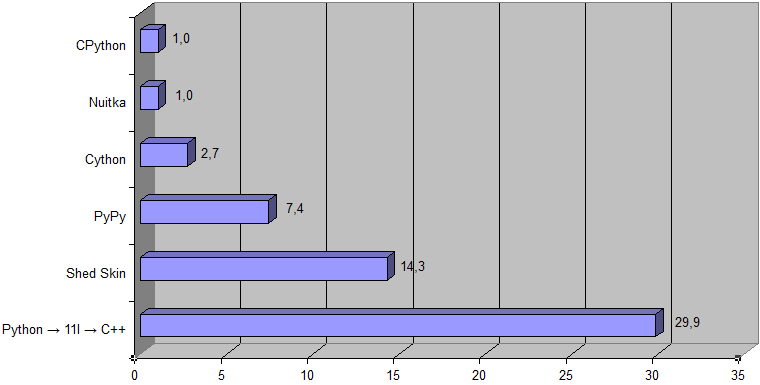``` ████ ██ ██ ██ ██ ██ ██ ██████████ ``` ``` ████ ██ ██ ██ ██ ██ ██ ██████████ ``` ``` ████ ██ ██ ██ ██ ██ ██ ██████████ ```

11l is a [semi-esoteric] compiled general-purpose programming language, which provides a simple and direct mapping to hardware and aims to follow a zero-overhead principle, like C++ (unused language features do not add run-time overhead). 11l is statically typed, but feels very much like a dynamically typed language (e.g. Python).
The design of 11l is aimed to achieve as much conciseness as possible.
Also 11l tries to combine a good code readability of Python {} with speed/performance of C++ {}.

The evident distinction of 11l from all other existing programming languages is its keywords are organized in a tree, not just a list.
There are 11 keywords at the root of that tree, and each word can be written as one letter. [Short one-letter keywords look odd at first sight, but it's just a matter of habit.]
Tree of all 11l keywords [including few not implemented yet]

Samples

 Performance The Python → 11l → C++ transpiler can be used to accelerate Python code. Here is results for pqmarkup to HTML converter (as an input data the source of this article is taken):(Shed Skin is not listed here because it does not support nested functions.) [Here is an archive with testing program used (Python 3.6 or higher is required, and following Python packages: pywin32, cython).] This is the results for a Prime number problem (with K = 1000000):Here is a corresponding Python source code ```import math k = int(input()) n = k * 17 primes = [True] * n primes = primes = False for i in range(2, int(math.sqrt(n)) + 1): if not primes[i]: continue for j in range(i * i, n, i): primes[j] = False for i in range(n): if primes[i]: if k == 1: print(i) break k -= 1 ``` And this code {… ```# Copyright (c) Project Nayuki. All rights reserved. Modified with permission. def is_right_triangle(x1, y1, x2, y2): a = x1**2 + y1**2 b = x2**2 + y2**2 c = (x2 - x1)**2 + (y2 - y1)**2 return (a + b == c) or (b + c == a) or (c + a == b) LIMIT = 51 ans = 0 for x1 in range(LIMIT): for y1 in range(LIMIT): for x2 in range(LIMIT): for y2 in range(LIMIT): # For uniqueness, ensure that (x1,y1) has a larger angle than (x2,y2) if y2 * x1 < y1 * x2 and is_right_triangle(x1, y1, x2, y2): ans += 1 print(ans) ``` (Based on this solution.) } for solving problem #91 of the Project Euler is ~500 times faster (the Python → 11l → C++ transpiler vs CPython):Download Here is the latest version of transpiler-pair Python → 11l and 11l → C++: 11l.tar.xz . Usage: Unpack archive to whatever directory you want. Open Terminal/cmd. Run command: `\11l ` on Windows, or `/11l ` on Linux. Having problems with compiling your Python or 11l program? Please read this guide. And if you haven't found a solution there, then write at the forum.